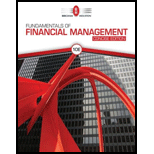# CONSTANT GROWTH VALUATION Holtzman Clothiers’s stock currently sells for $38.00 a share. It just paid a dividend of$2.00 a share (i.e., D 0 = $2.00). The dividend is expected to grow at a constant rate of 5% a year. What stock price is expected 1 year from now? What is the required rate of return?BuyFindarrow_forward ### Fundamentals Of Financial Manageme... 10th Edition Eugene F. Brigham + 1 other Publisher: Cengage Learning ISBN: 9781337902571 #### Solutions Chapter SectionBuyFindarrow_forward ### Fundamentals Of Financial Manageme... 10th Edition Eugene F. Brigham + 1 other Publisher: Cengage Learning ISBN: 9781337902571 Chapter 9, Problem 3P Textbook Problem 12 views ## CONSTANT GROWTH VALUATION Holtzman Clothiers’s stock currently sells for$38.00 a share. It just paid a dividend of $2.00 a share (i.e., D0 =$2.00). The dividend is expected to grow at a constant rate of 5% a year. What stock price is expected 1 year from now? What is the required rate of return?

Summary Introduction

To determine: The expected stock price after 1 year from now and the required rate of return.

Introduction:

Dividends per Share: The periodic rewards that are received by the stockholders for their investment in a company are known as dividends. It is also a measure based on per share. Per share, calculation of dividends is called as the dividends per share.

### Explanation of Solution

Compute next year expected price, ( P1 ).

Given,

Current stock price ( P0 ) is $38 per share. Growth rate (g) is 5% or 0.05. The formula to calculate next expected price is, P1=P0(1+g) Where, • P0 is the current stock price. • g is the growth rate. • P1 is the next expected price. Substitute,$38 for P0 and 0.05 for g

P1=$38(1+0.05)=$38×1.05=$39.9 The expected price of the stock for the next year is$39.9.

Compute the required rate of return.

Given,

Current stock price ( P0 ) is $38 per share. Growth rate (g) is 5% or 0.05. Current dividend paid ( D0 ) is$2

### Still sussing out bartleby?

Check out a sample textbook solution.

See a sample solution

#### The Solution to Your Study Problems

Bartleby provides explanations to thousands of textbook problems written by our experts, many with advanced degrees!

Get Started

Find more solutions based on key concepts
Why are there so many laws relating to HRM practices?

Foundations of Business (MindTap Course List)

RATIO ANALYSIS Data for Barry Computer Co. and its industry averages follow. a. Calculate the indicated ratios ...

Fundamentals of Financial Management, Concise Edition (with Thomson ONE - Business School Edition, 1 term (6 months) Printed Access Card) (MindTap Course List)

Leverage is the ability to pay debts when they come due.

College Accounting, Chapters 1-27 (New in Accounting from Heintz and Parry)